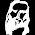## Saturday, June 15, 2013

### Problem 889: Carnot's Theorem in an acute triangle, Circumcenter, Circumradius, Inradius

Geometry Problem. GeoGebra, HTML5 Animation for iPad and Nexus.

Level: Mathematics Education, High School, Honors Geometry, College.

Click the figure below to see the dynamic geometry demostration of problem 889.1.OA1 + OB1 + OC1
= R (cosA + cosB + cosC)
= R (1 + 4 sinA/2 sinB/2 sinC/2)
= R + 4R sinA/2 sinB/2 sinC/2
= R + r

2.The last step follows from

r (cotB/2 + cotC/2) = a = 2R sinA = 4R sinA/2 cosA/2
r = 4R sinA/2 sinB/2 sinC/2